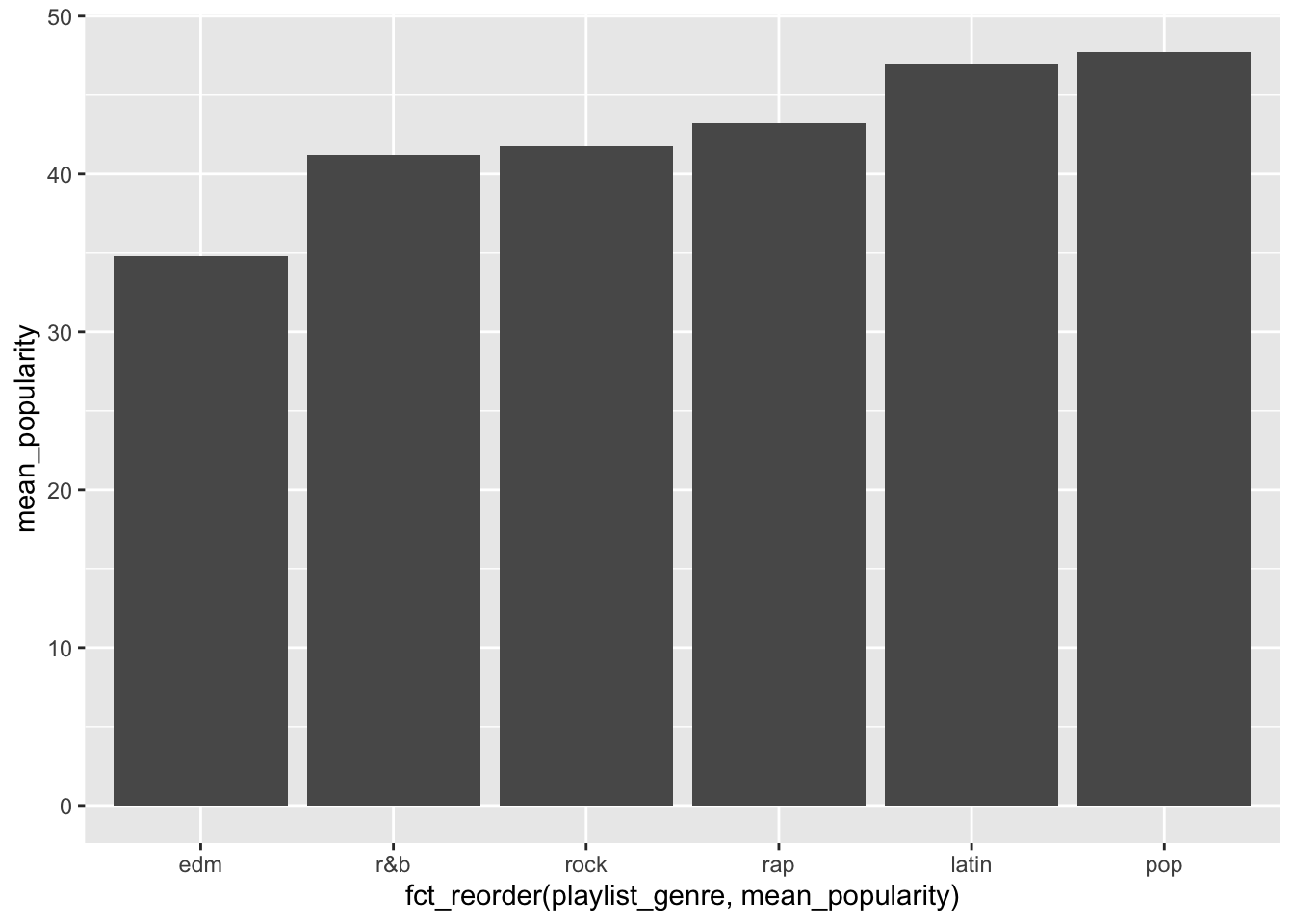# Module 8 Data Visualization

## 8.1 The layered grammar of graphics

The package we will be using for plotting in this class is called `ggplot2` which is part of `tidyverse`, and it uses as a principle the idea of layered grammar of graphics. That means you can add code to add or change your plot in layers, by using the `+` symbol.

Let’s start with some data, so we can created different plots using different layers.

## 8.2 Load data

Get data directly from tidy tuesday github.

``````## Rows: 32,833
## Columns: 23
## \$ track_id                 <chr> "6f807x0ima9a1j3VPbc7VN", "0r7CVbZTWZgbTCYdf…
## \$ track_name               <chr> "I Don't Care (with Justin Bieber) - Loud Lu…
## \$ track_artist             <chr> "Ed Sheeran", "Maroon 5", "Zara Larsson", "T…
## \$ track_popularity         <dbl> 66, 67, 70, 60, 69, 67, 62, 69, 68, 67, 58, …
## \$ track_album_id           <chr> "2oCs0DGTsRO98Gh5ZSl2Cx", "63rPSO264uRjW1X5E…
## \$ track_album_name         <chr> "I Don't Care (with Justin Bieber) [Loud Lux…
## \$ track_album_release_date <chr> "2019-06-14", "2019-12-13", "2019-07-05", "2…
## \$ playlist_name            <chr> "Pop Remix", "Pop Remix", "Pop Remix", "Pop …
## \$ playlist_id              <chr> "37i9dQZF1DXcZDD7cfEKhW", "37i9dQZF1DXcZDD7c…
## \$ playlist_genre           <chr> "pop", "pop", "pop", "pop", "pop", "pop", "p…
## \$ playlist_subgenre        <chr> "dance pop", "dance pop", "dance pop", "danc…
## \$ danceability             <dbl> 0.748, 0.726, 0.675, 0.718, 0.650, 0.675, 0.…
## \$ energy                   <dbl> 0.916, 0.815, 0.931, 0.930, 0.833, 0.919, 0.…
## \$ key                      <dbl> 6, 11, 1, 7, 1, 8, 5, 4, 8, 2, 6, 8, 1, 5, 5…
## \$ loudness                 <dbl> -2.634, -4.969, -3.432, -3.778, -4.672, -5.3…
## \$ mode                     <dbl> 1, 1, 0, 1, 1, 1, 0, 0, 1, 1, 1, 1, 1, 0, 0,…
## \$ speechiness              <dbl> 0.0583, 0.0373, 0.0742, 0.1020, 0.0359, 0.12…
## \$ acousticness             <dbl> 0.10200, 0.07240, 0.07940, 0.02870, 0.08030,…
## \$ instrumentalness         <dbl> 0.00e+00, 4.21e-03, 2.33e-05, 9.43e-06, 0.00…
## \$ liveness                 <dbl> 0.0653, 0.3570, 0.1100, 0.2040, 0.0833, 0.14…
## \$ valence                  <dbl> 0.518, 0.693, 0.613, 0.277, 0.725, 0.585, 0.…
## \$ tempo                    <dbl> 122.036, 99.972, 124.008, 121.956, 123.976, …
## \$ duration_ms              <dbl> 194754, 162600, 176616, 169093, 189052, 1630…``````

EXERCISE

1. What variables do we have in this data?

## 8.3 What to plot?

The first thing you need to do is define what you want to plot. If you’ve never plotted data before, you might not be familiar with the different types of charts you can create.

Here’s a few (can you tell what type of plot these are?):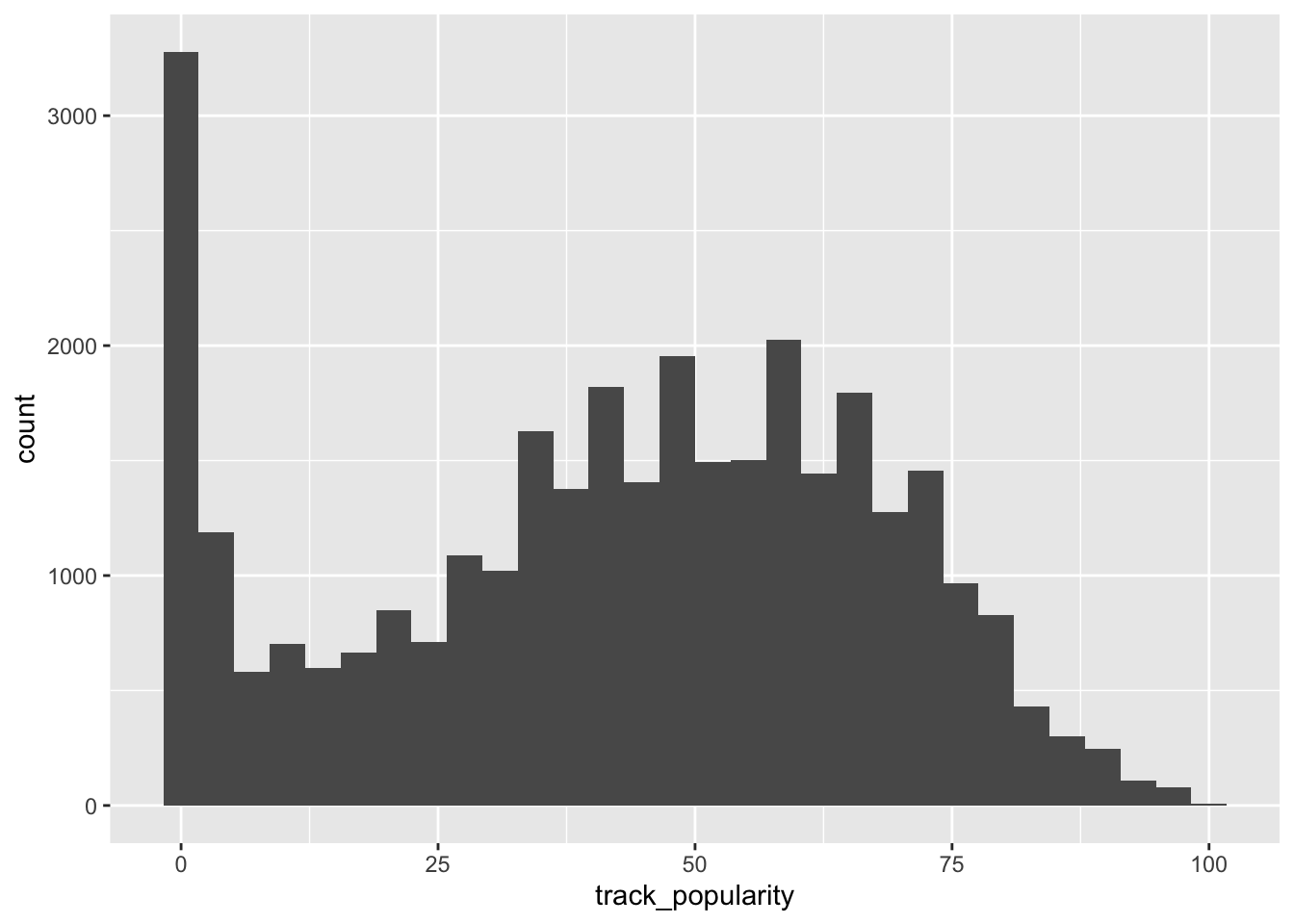1. What is the plot above called?

2. What variable(s) are we plotting?

3. What can we conclude based on this plot?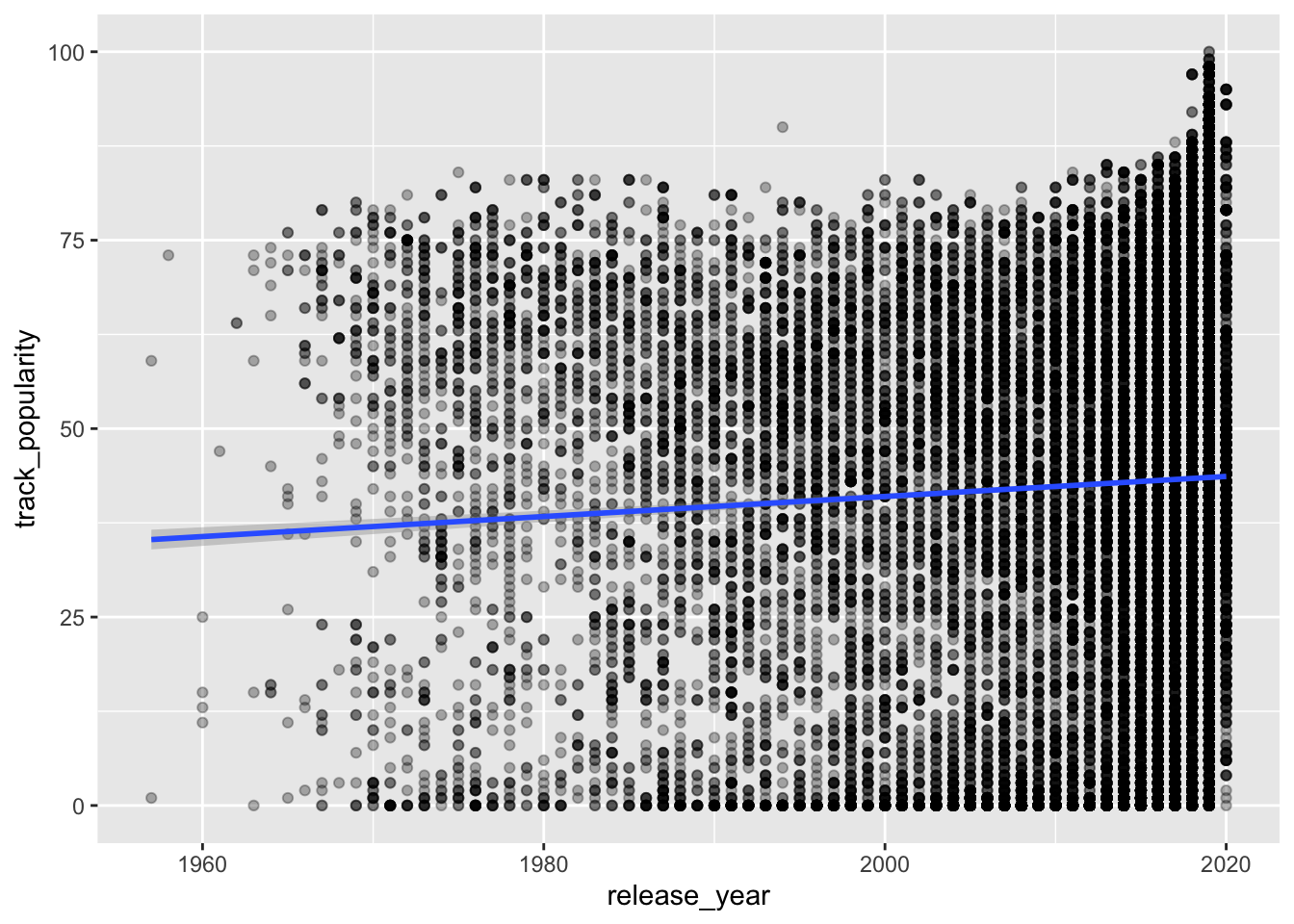1. What is the plot above called?

2. What variable(s) are we plotting?

3. What can we conclude based on this plot?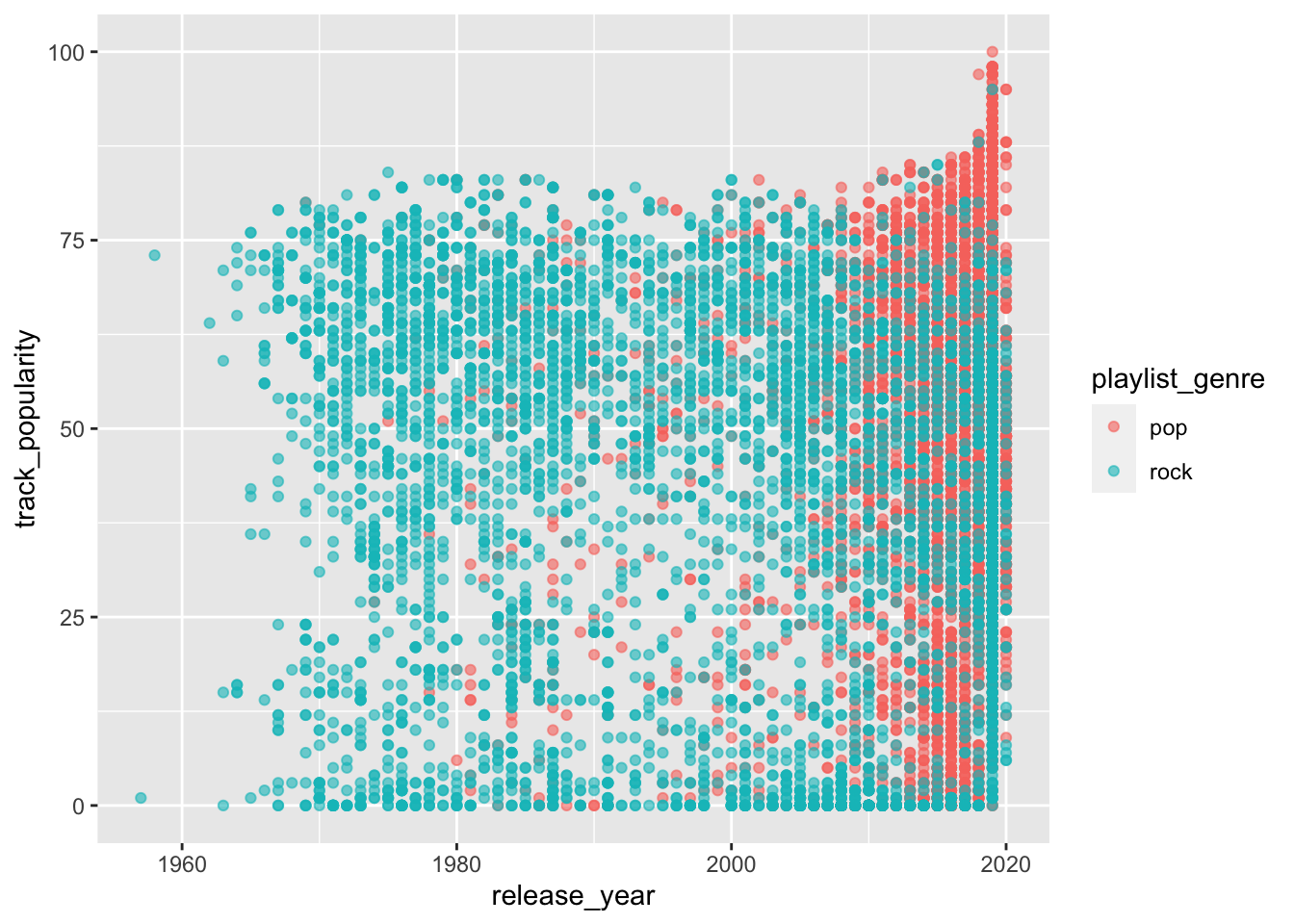1. What is the plot above called?

2. What variable(s) are we plotting?

3. What can we conclude based on this plot?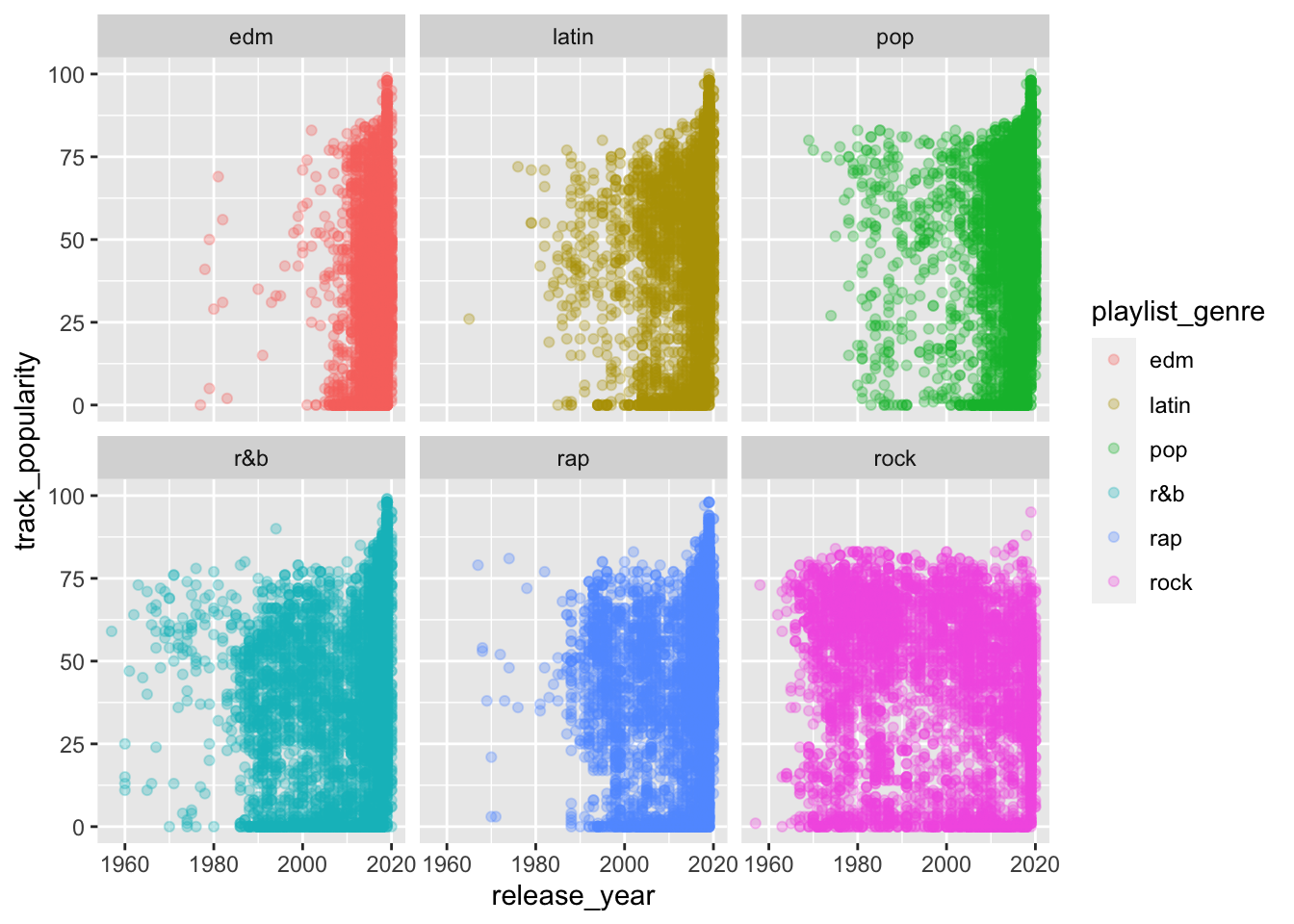1. What is the plot above called?

2. What variable(s) are we plotting?

3. What can we conclude based on this plot?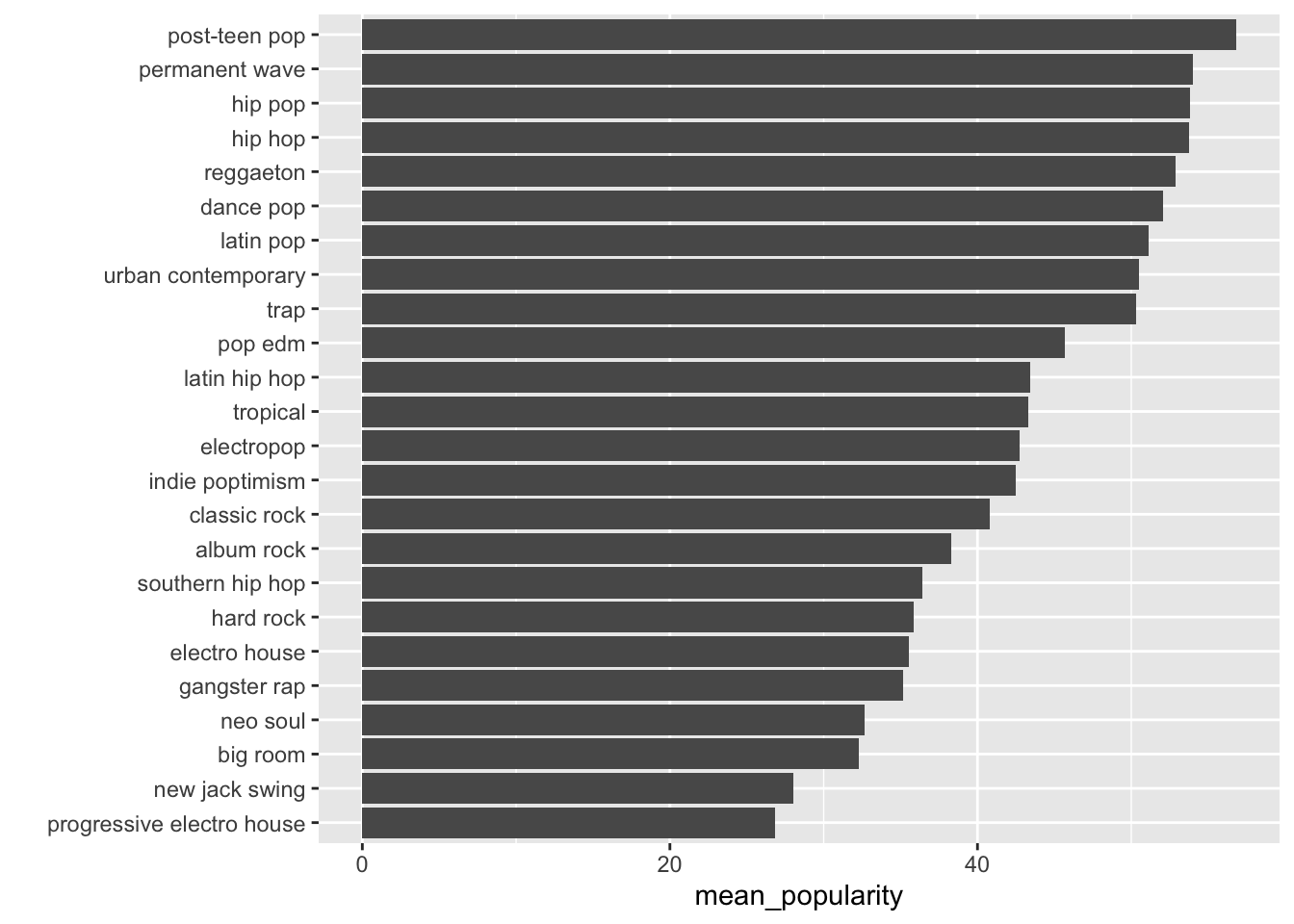1. What is the plot above called?

2. What variable(s) are we plotting?

3. What can we conclude based on this plot?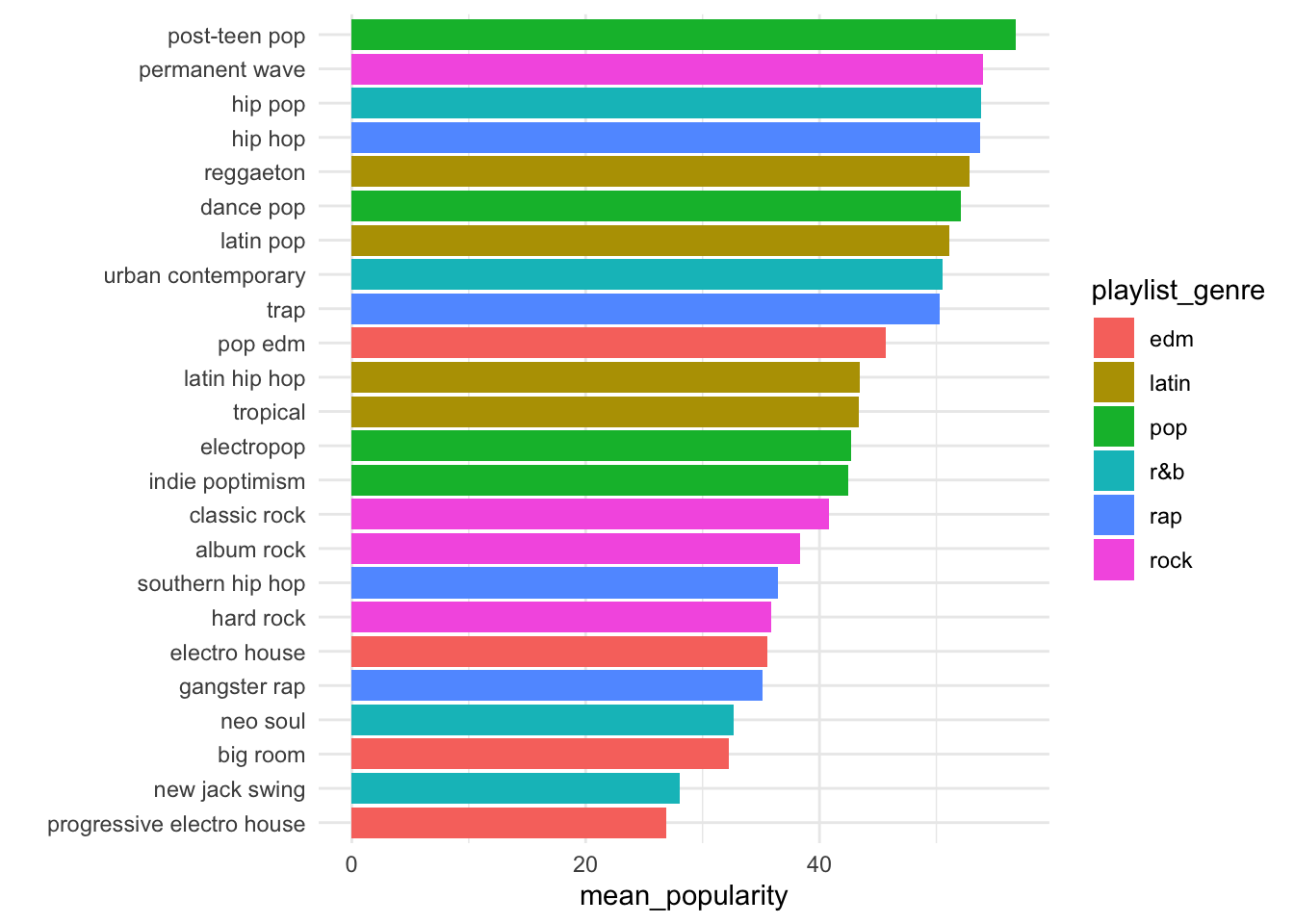1. What is the plot above called?

2. What variable(s) are we plotting?

3. What can we conclude based on this plot?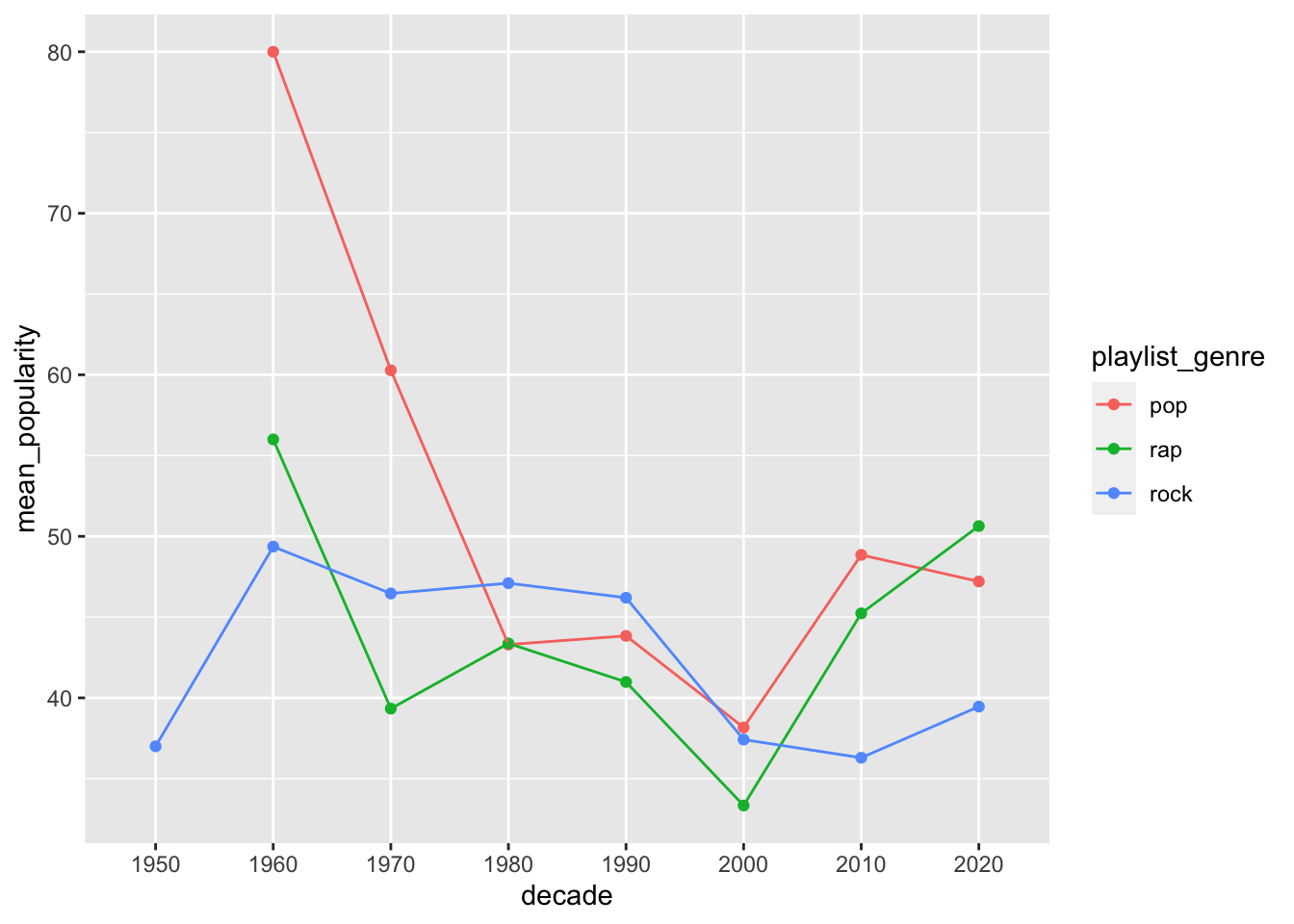## 8.4 Aesthetic Mappings

You map your aesthetics using the `aes()` function, which can be place inside of the `ggplot()` function.

### 8.4.1 One continuous variable

You need to map at least one variable when you are plotting. Check the help for `geom_histogram` to see what kind of variable you can plot in a histogram.

The variable `track_popularity` is continuous.

``````spotify_songs %>%
ggplot(aes(x = track_popularity)) +
geom_histogram() ``````
``## `stat_bin()` using `bins = 30`. Pick better value with `binwidth`.``The variable `release_year` is also continuous.

``````spotify_songs %>%
ggplot(aes(x = release_year)) +
geom_histogram() ``````
``## `stat_bin()` using `bins = 30`. Pick better value with `binwidth`.``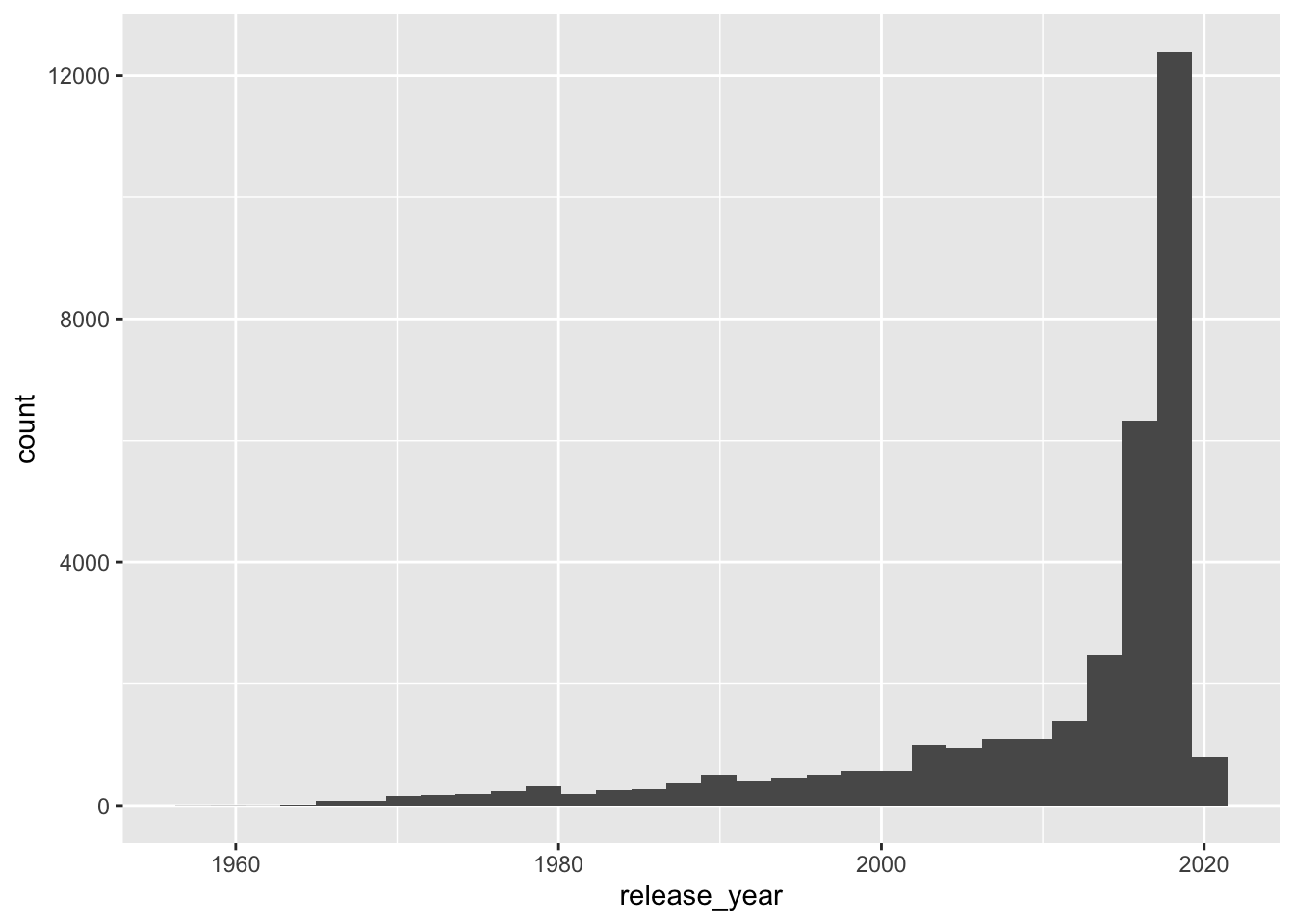### 8.4.2 Two numeric variables

Two-dimensional plots have two axis, `x` (horizontal) and `y` (vertical).

``````spotify_songs %>%
ggplot(aes(x = release_year,
y = track_popularity)) +
geom_point() ``````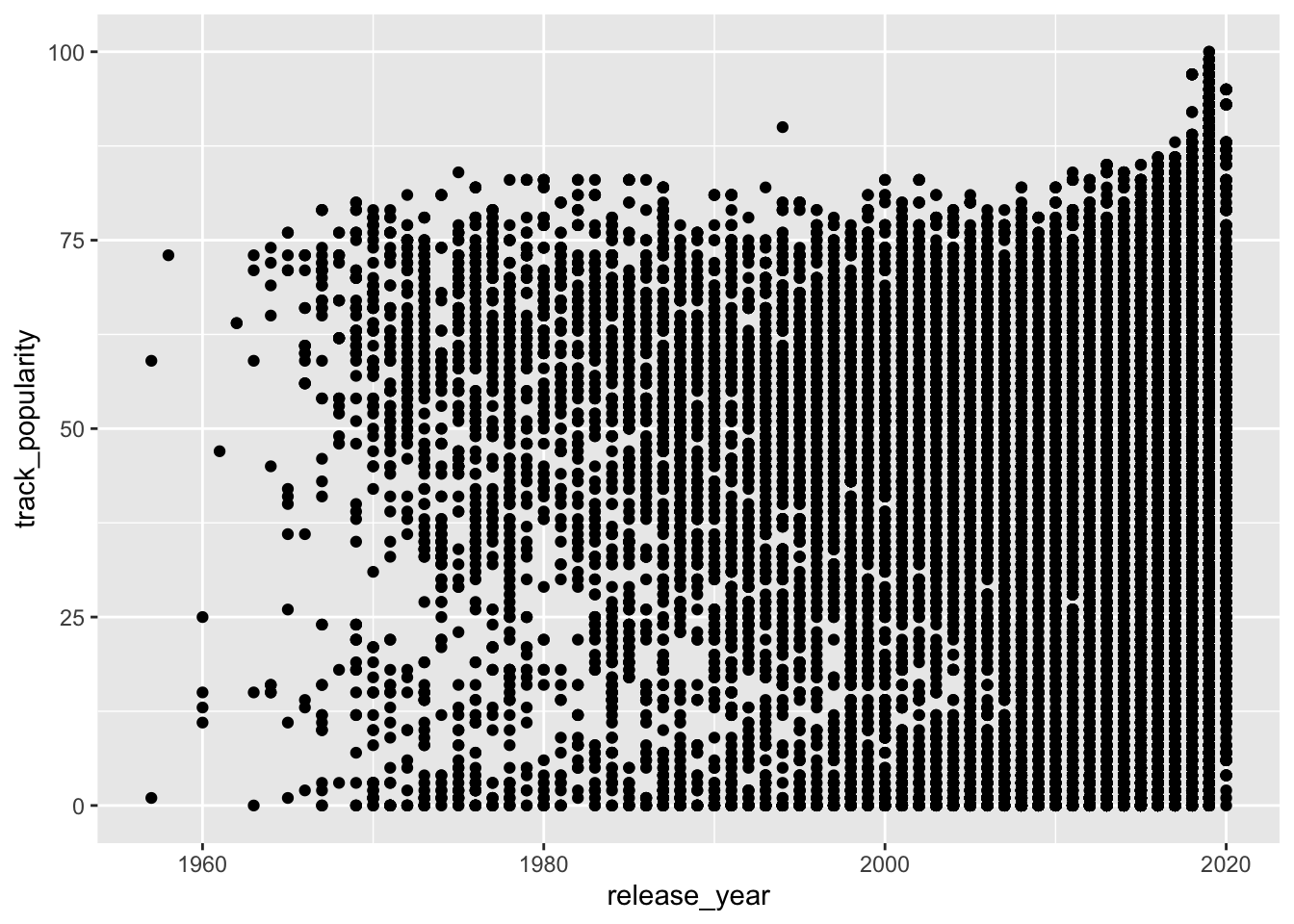## 8.5 geom_ (i.e., Geometric Objects)

Functions such as `geom_histogram()`, `geom_point()`, and `geom_col()` are geometric objects and determine what type of plot R draws.

You can also map other elements of your chart in addition to position (i.e., `x` and `y`), such as color, size, and shape.

``````spotify_songs %>%
ggplot(aes(x = release_year,
y = track_popularity,
color = playlist_genre)) +
geom_point() ``````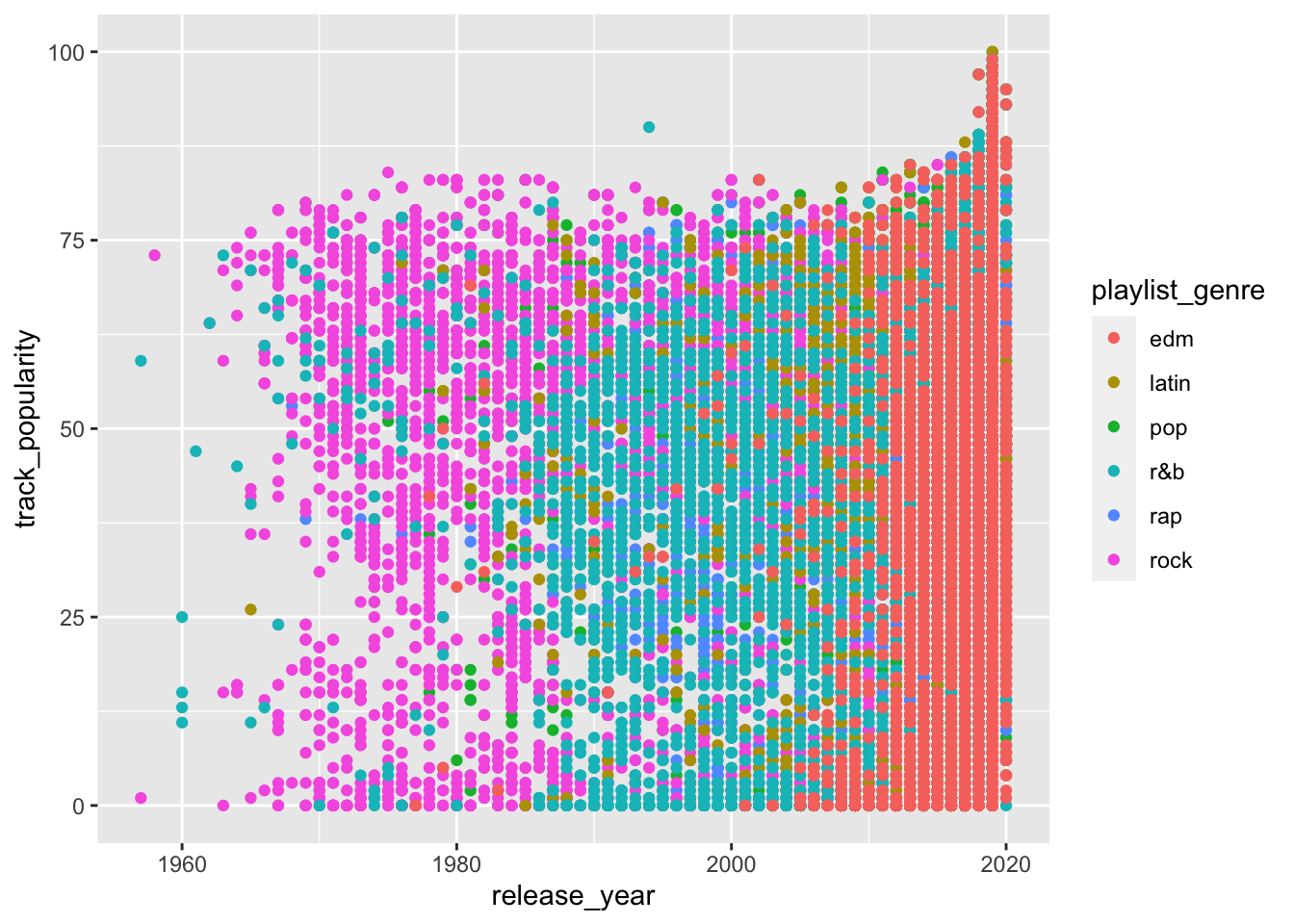EXERCISE

Check the help page for `geom_point` (enter `?geom_point` in your console). Change `geom_point()` to the suggested variations in its help page.

## 8.6 More mappings with aes()

In addition to `color` you can also add `size` and `shape` to `aes()`.

``````spotify_songs %>%
ggplot(aes(x = release_year,
y = track_popularity,
color = playlist_subgenre,
shape = playlist_genre)) +
geom_point() ``````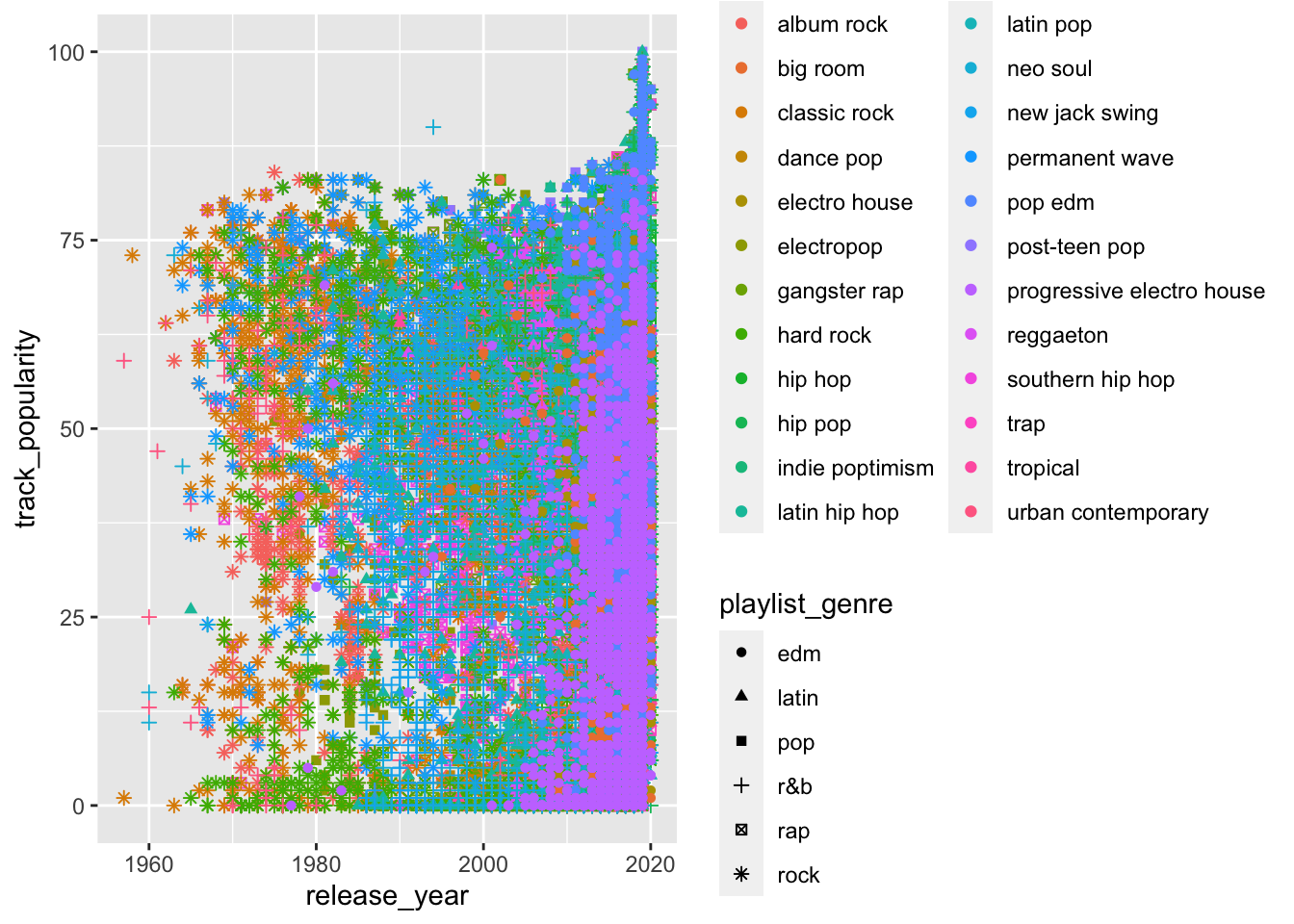The plot above is too messy, there’s too much information. You often need to transform your data before plotting it.

EXERCISE

Summarize mean `track_popularity` by `release_year`, `playlist_genre` and `playlist_subgenre`.

Your summarized data frame should look something like this:

``## `summarise()` regrouping output by 'release_year', 'playlist_genre' (override with `.groups` argument)``
``````## # A tibble: 883 x 4
## # Groups:   release_year, playlist_genre 
##    release_year playlist_genre playlist_subgenre  mean_popularity
##           <dbl> <chr>          <chr>                        <dbl>
##  1         1957 r&b            urban contemporary              59
##  2         1957 rock           classic rock                     1
##  3         1958 rock           classic rock                    73
##  4         1960 r&b            neo soul                        13
##  5         1960 r&b            urban contemporary              19
##  6         1961 r&b            urban contemporary              47
##  7         1962 r&b            urban contemporary              64
##  8         1962 rock           classic rock                    64
##  9         1963 r&b            neo soul                        73
## 10         1963 rock           album rock                      59
## # … with 873 more rows``````

Now you can plot your summarized data.

``````spotify_summary %>%
ggplot(aes(x = release_year,
y = mean_popularity,
color = playlist_subgenre,
shape = playlist_genre)) +
geom_point() ``````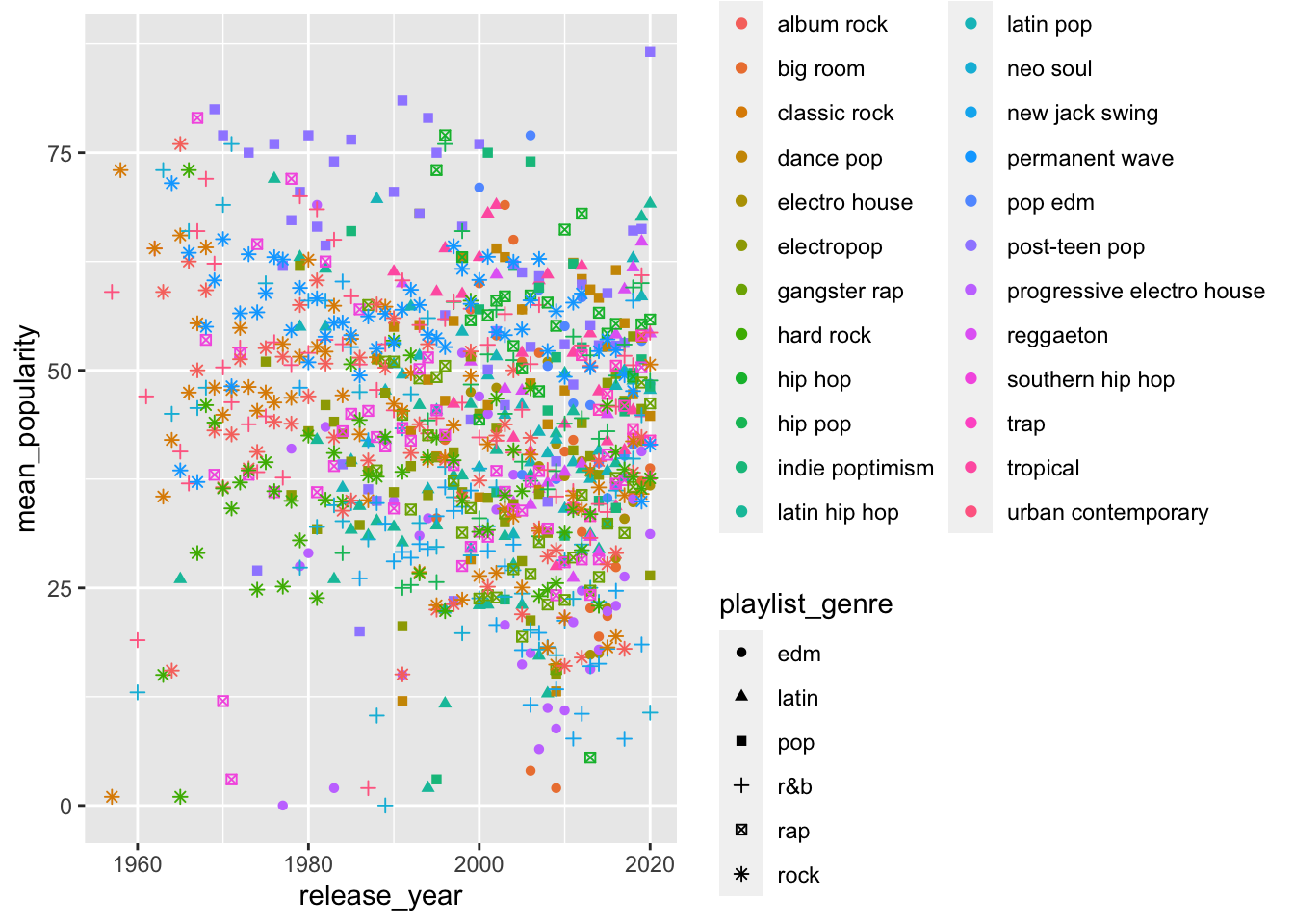Still super messy. Shape is not really a good way to do this.

Let’s try a bar plot.

``````spotify_summary %>%
ggplot(aes(x = release_year,
y = mean_popularity,
fill = playlist_genre)) +
geom_col(position = "dodge") ``````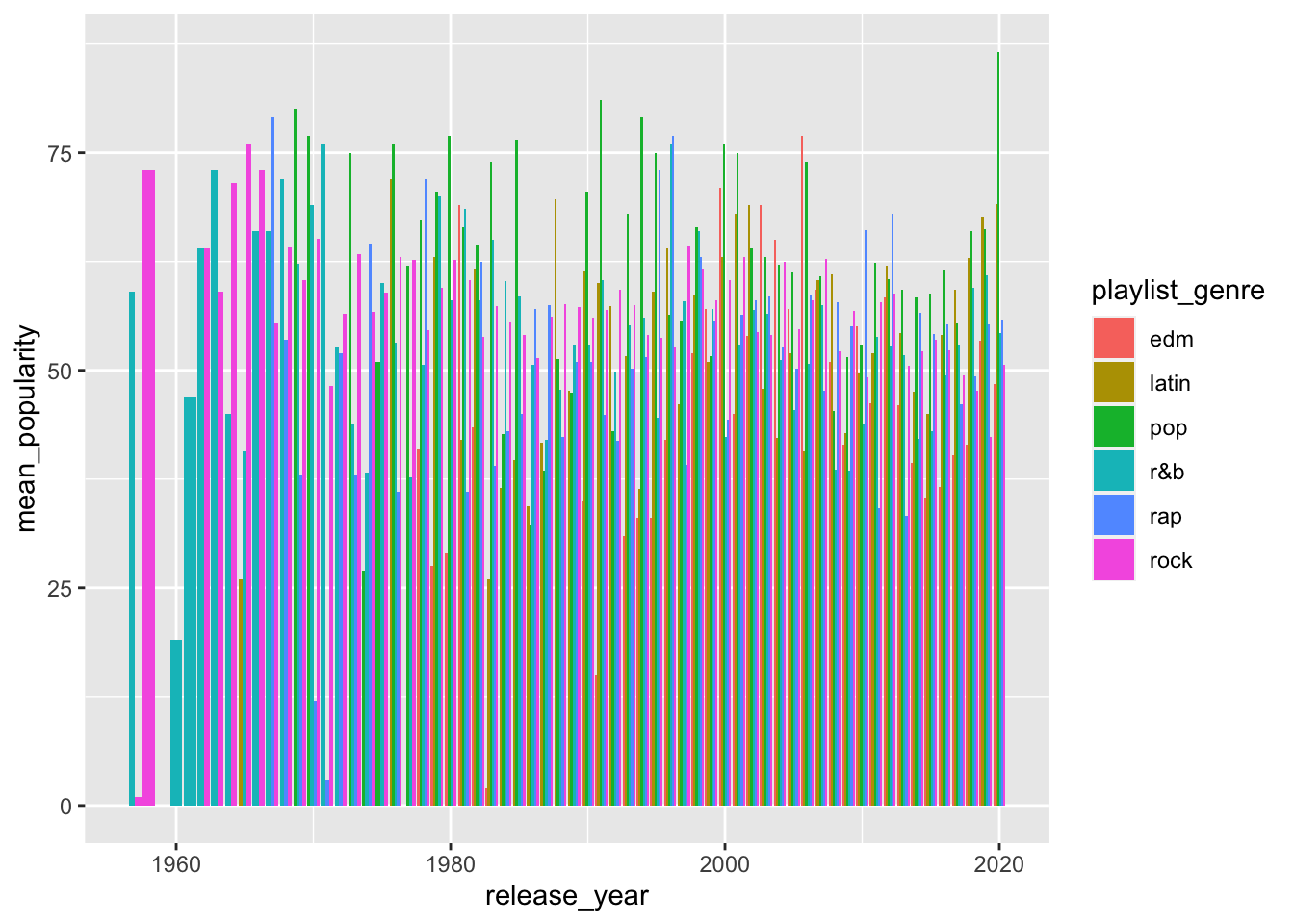Not great either, too much going on.

## 8.7 Facets

You can use the `facet_wrap()` function to split your plotting into several smaller plots, usually by a categorical variable.

Let’s try the scatterplot first.

``````spotify_summary %>%
ggplot(aes(x = release_year,
y = mean_popularity,
color = playlist_genre)) +
geom_point() +
facet_wrap(~playlist_subgenre)``````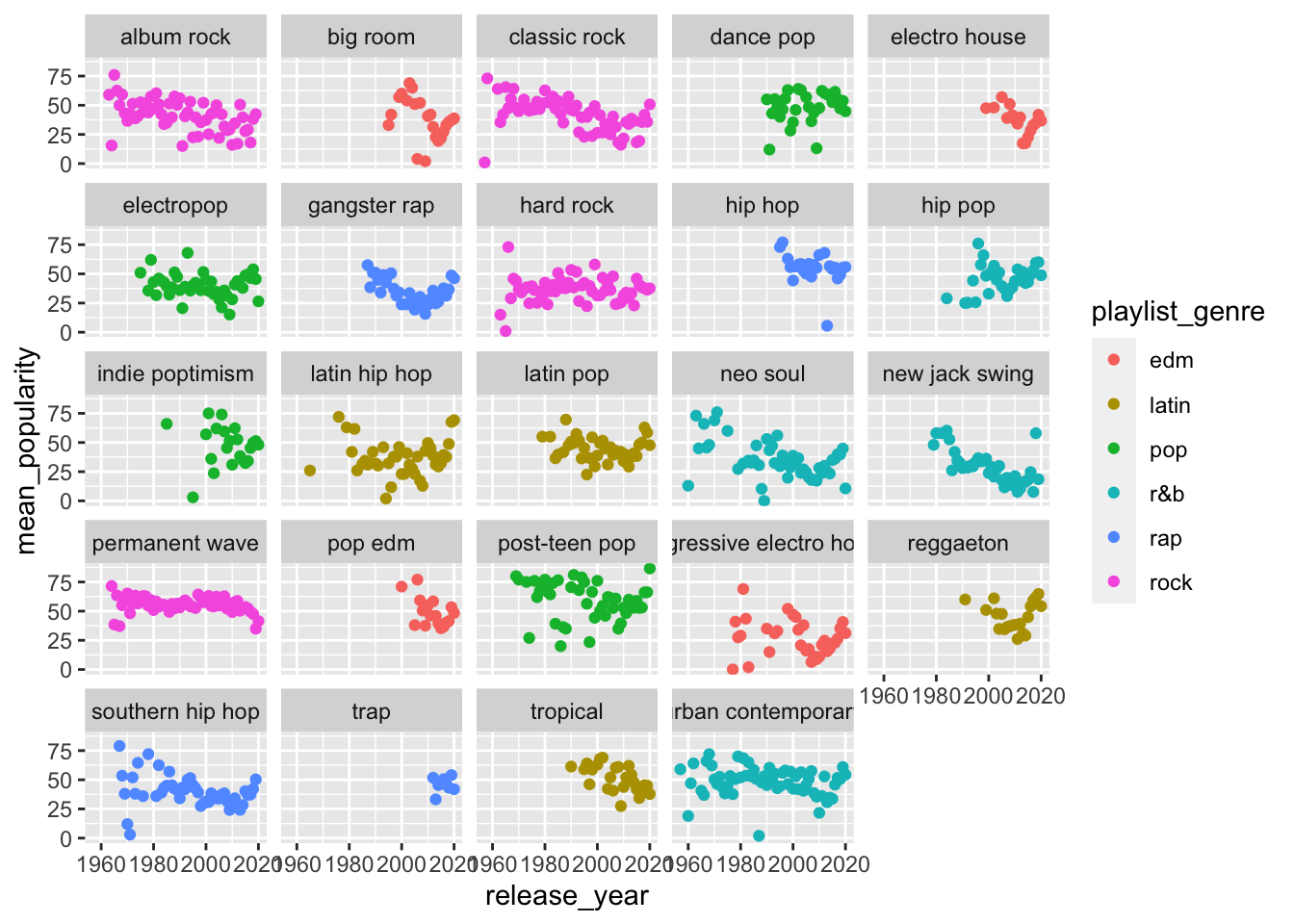What about a bar plot?

``````spotify_summary %>%
ggplot(aes(x = release_year,
y = mean_popularity,
fill = playlist_genre)) +
geom_col(position = "dodge") +
facet_wrap(~playlist_subgenre)``````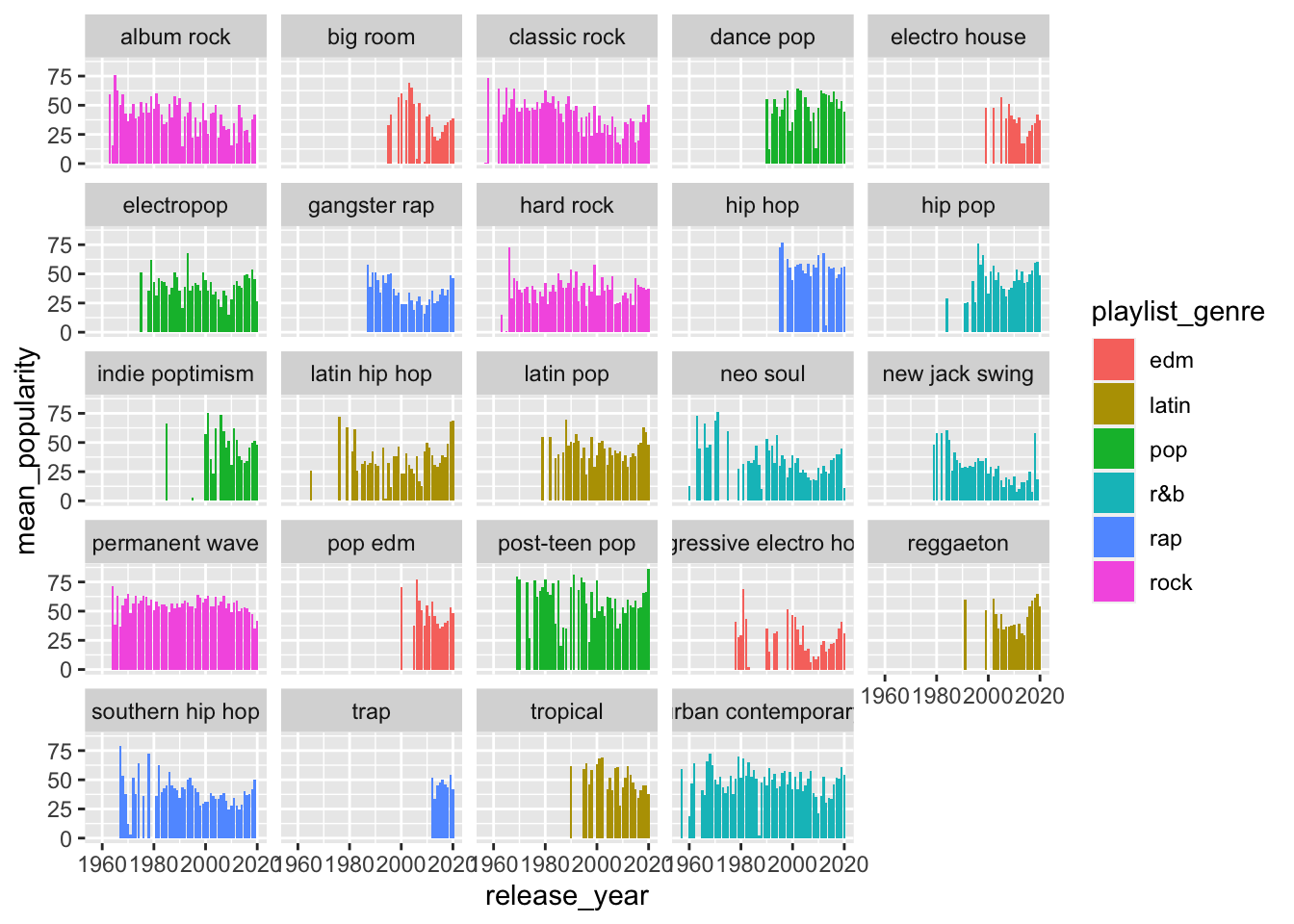## 8.8 More Summarize

We can also plot categorical variables on the `x` axis.

EXERCISE

Summarize mean `track_popularity` by `playlist_genre`.

Your summarized data frame should look something like this:

``## `summarise()` ungrouping output (override with `.groups` argument)``
``````## # A tibble: 6 x 2
##   playlist_genre mean_popularity
##   <chr>                    <dbl>
## 1 edm                       34.8
## 2 latin                     47.0
## 3 pop                       47.7
## 4 r&b                       41.2
## 5 rap                       43.2
## 6 rock                      41.7``````

Now plot a bar chart mapping `x` to `playlist_genre`, `y` to `mean_popularity`.

Your plot should look something like this: